# JEE Main 2020 Paper with Solutions Physics 9th Jan Shift 2

JEE is an examination conducted for admission into some of the reputed organisations across the country. The examination is conducted in two levels - JEE Main and JEE Advanced. The subjects for the engineering entrance tests are Mathematics, Physics and Chemistry. The questions are based on Class 11 and Class 12 syllabus. Candidates can find answers for all the questions asked in JEE Main 2020 Physics Paper (Shift 2 - 9th January) on this page.

### January 9 Shift 2 - Physics

1.An electron gun is placed inside a long solenoid of radius R on its axis. The solenoid has n turns/length and carries a current i. The electron gun shoots an electron along the radius of solenoid with speed If the electron does not hit the surface of the solenoid, maximum possible value of v is (all symbols have their standard meaning):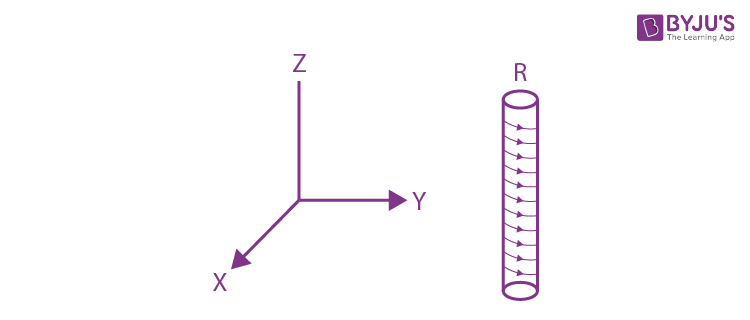1. a.
$$\frac{2Re\mu _{0}in}{m}$$
2. b.
$$\frac{Re\mu _{0}in}{2m}$$
3. c.
$$\frac{Re\mu _{0}in}{m}$$
4. d.
$$\frac{Re\mu _{0}in}{4m}$$

Solution:

1. Looking at the cross-section of the solenoid, Rmax of the particle’s motion has to be R/2 for it not to strike the solenoid.$$evB = mv^{2}/R$$

$$R_{max}=\frac{R}{2}=\frac{mv_{max}}{e\mu _{0}in}$$

$$V_{max}=\frac{Re\mu _{0}in}{2m}$$

2.Two identical capacitors A and B, charged to the same potential 5V are connected in two different circuit as shows below at time t=0. If the charges on capacitors A and B at time t= CR is QA and QB respectively, then (Here is the base of natural logarithm)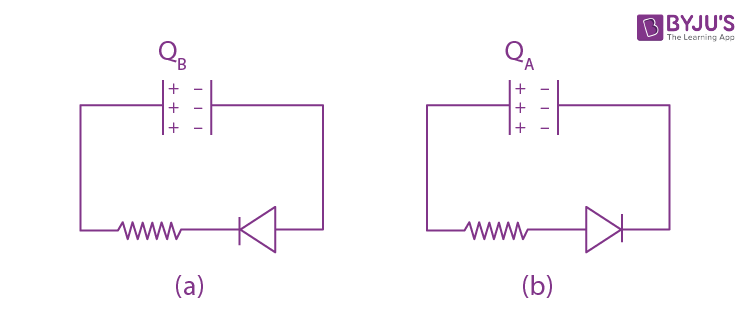1. a. CV,CV/e
2. b. CV/e, CV/2e
3. c. CV/e, CV/2
4. d. CV/e, CV

Solution:

1. For (a)

No current flows

Hence, QA = CV

For (b)

Current will flow in the circuit

I = (V/R)e(t/RC)

Charge on the capacitor at any time q = Q e(-t/RC)

at t =CR

q = Q e(-CR/RC)

q = Q e-1= Q/e

q = CV/e= QB

3. For the four sets of three measured physical quantities as given below. Which of the following options is correct?

(i) A1 = 24.36, B1=0.0724, C1= 256.2

(ii) A2 = 24.44, B2=16.08, C2= 240.2

(iii) A3 = 25.2, B3 = 19.2812, C3= 236.183

(iv) A4 = 25, B4 = 236.191, C4= 19.5

1. a. A4 + B4 +C4 < A1 + B1 +C1< A2 + B2 +C2= A3 + B3 +C3
2. b. A1 + B1 +C1= A2 + B2 +C2=A3 + B3 +C3= A4 + B4 +C4
3. c. A1 + B1 +C1< A3 + B3 +C3< A2 + B2 +C2< A4 + B4 +C4
4. d. A4 + B4 +C4 < A1 + B1 +C1< A3 + B3 +C3< A2 + B2 +C2

Solution:

1. A1 + B1 +C1= 280.632

A2 + B2 +C2= 280.72

A3 + B3 +C3 = 280.664

A4 + B4 +C4 = 280.691

Hence, option (a) is correct.

4.A particle starts from the origin at t = 0 with an initial velocity of

$$\vec{u}=3\hat{i}$$
from origin and moves in the x-y plane with a constant acceleration
$$\vec{a}=6\hat{i}+4\hat{j}m/s^{2}$$
. The x- coordinate of the particle at the instant when its y-cordinated is 32 meters. The value of D is:

1. a. 60
2. b. 50
3. c. 32
4. d. 40

Solution:

1. $$S_{y}=u_{y}t+\frac{1}{5}a_{y}t^{2}$$

$$32=0 +\frac{1}{2}4t^{2}$$

t= 4 sec

$$S_{x}=u_{x}t+\frac{1}{5}a_{x}t^{2}$$

= 3 x 4 + (1/2) x 6 x 16

= 60 m

5. A spring mass system (mass m, spring constant k and natural length l) rest in equilibrium on a horizontal disc. The free end of the spring is fixed at the center of the disc. If the disc together with spring mass system, rotates about its axis with an angular velocity (k>>>m2), the relative change in the length of the spring is best given by the option:

1. a.
$$\frac{m\omega^{2} }{3k}$$
2. b .
$$\sqrt{\frac{2}{3}}\frac{m\omega^{2} }{k}$$
3. c.
$$\frac{m\omega^{2} }{k}$$
4. d .
$$\frac{2m\omega^{2} }{k}$$

Solution:

1.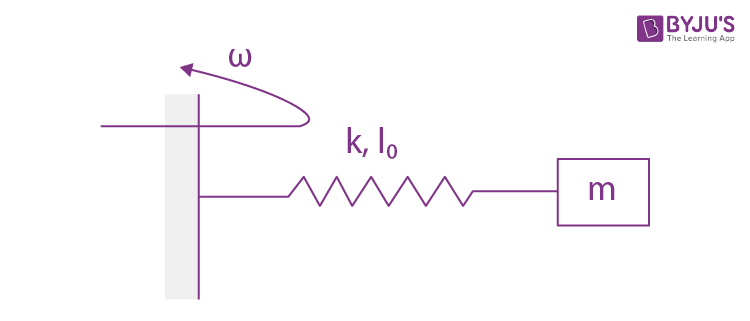Using Newton’s second law of dynamics,

$$m\omega^{2}(l_{0}+x)=kx$$

$$(\frac{l_{0}}{x}+1)=\frac{kx}{m\omega^{2}}$$

$$x= (\frac{l_{0}m\omega^{2}}{k-m\omega^{2}})$$

k>>mω2

So, (x/l0) is equal to mω2/k

6. A small circular loop of conducting wire has radius a and carries current i. It is placed in a uniform magnetic field B perpendicular to its plane such that when rotated slightly about its diameter and released, its starts performing simple harmonic motion of time period T. If the mass of the loop is m then

1. a.
$$T= \sqrt{\frac{\pi M}{iB}}$$
2. b.
$$T= \sqrt{\frac{2\pi M}{iB}}$$
3. c.
$$T= \sqrt{\frac{\pi M}{2iB}}$$
4. d.
$$T= \sqrt{\frac{2M}{iB}}$$

Solution:

1.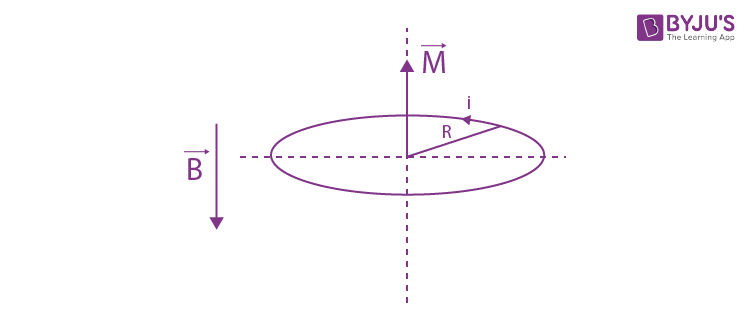Considering the torque situation on the loop,

$$\tau =MBsin\theta = -I\alpha$$

$$\pi R^{2}iB\theta =\frac{mR^{2}}{2}\alpha$$

The above equation is analogous to

$$\theta =-C\alpha$$
where

$$C= \omega ^{2}=2\pi iB/M$$

$$\omega =\sqrt{2\pi iB/M}=2\pi /T$$

$$T = \sqrt{\frac{2\pi M}{iB}}$$

7. A small spherical droplet of density d is floating exactly half immersed in a liquid of density ρ and surface tension T. The radius of droplet is (take note that the surface tension applied an upward force on droplet)

1. a.
$$r= \sqrt{\frac{2T}{3(\rho +d)g}}$$
2. b.
$$r= \sqrt{\frac{T}{(\rho +d)g}}$$
3. c.
$$r= \sqrt{\frac{T}{(d-\rho )g}}$$
4. d.
$$r= \sqrt{\frac{3T}{(2\rho- d)g}}$$

Solution:

1.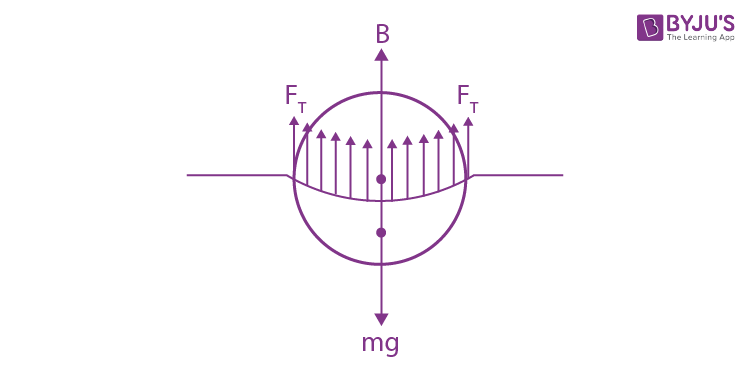In equilibrium, net external force acting on the sphere is zero.

Mg = FT+B

$$\rho Vg=d(V/2)g+T2\pi R$$

$$\rho\frac{4}{3}\pi R^{3}g=d\frac{2}{3}\pi R^{3}g+T2\pi R$$

$$r= \sqrt{\frac{3T}{(2\rho-d)g}}$$

8. A wire of length L and mass 6 x 10-3 kg/m per unit length is put under tension of 540N. Two consecutive frequencies that it resonates at are: 420 Hz and 490 Hz . Then L in meter is

1. a. 8.1 m
2. b. 2.1 m
3. c. 1.1 m
4. d. 5.1 m

Solution:

1. Key Idea: The difference of two consecutive resonant frequencies is the fundamental resonant frequency. Fundamental frequency = 490 – 420 =70 Hz

$$70 = \frac{1}{2l}\sqrt{\frac{T}{\mu }}$$

$$70 = \frac{1}{2l}\sqrt{\frac{540}{6\times 10^{-3} }}$$

l≈2.1 m

9. A plane electromagnetic wave is propagating along the direction

$$\frac{\hat{i}+\hat{j}}{\sqrt{2}}$$
with the polarization along the direction ̂
$$\hat{k}$$
.The correct form of the magnetic field of the wave would be ( here B0 is an appropriate constant)

1. a.
$$B_{0}\frac{\hat{i}-\hat{j}}{\sqrt{2}}cos(\omega t-k(\frac{\hat{i}+\hat{j}}{\sqrt{2}}))$$
2. b.
$$B_{0}\frac{\hat{i}+\hat{j}}{\sqrt{2}}cos(\omega t+k(\frac{\hat{i}+\hat{j}}{\sqrt{2}}))$$
3. c.
$$B_{0}\frac{\hat{i}-\hat{j}}{\sqrt{2}}cos(\omega t+k(\frac{\hat{i}+\hat{j}}{\sqrt{2}}))$$
4. d.
$$B_{0}\hat{k}cos(\omega t-k(\frac{\hat{i}+\hat{j}}{\sqrt{2}}))$$

Solution:

1. EM wave is in direction

$$\frac{\hat{i}+\hat{j}}{\sqrt{2}}$$

Electric field is in direction

$$\hat{k}$$

Direction of propagation of EM wave is given by

$$\vec{E}\times \vec{B}$$

10. Two gases-Argon (atomic radius 0.07 nm atomic weight 40) and Xenon (atomic radius 0.1 nm atomic weight 140) have the same number density and are at the same temperature. The ratio of their respective mean free time is closest to

1. a. 4.67
2. b. 2.04
3. c. 1.83
4. d. 3.67

Solution:

1. $$\lambda =\frac{1}{\sqrt{2\pi }n_{v}d^{2}}$$

$$\tau =\lambda /v$$

$$\frac{\tau _{1}}{\tau _{2}}=\frac{\sqrt{M_{1}}}{d_{1}^{2}}\times \frac{d_{2}^{2}}{\sqrt{M_{2}}}$$

$$\frac{\tau _{1}}{\tau _{2}}=\frac{\sqrt{40}}{0.07^{2}}\times \frac{0.1^{2}}{\sqrt{140}}$$

= 1.09

11. Two steel wires having same length are suspended from a ceiling under the same load. If the ratio of their energy stored per unit volume is 1: 4, the ratio of their diameters is:

1. a.√2:1
2. b.1: √2
3. c.1:2
4. d. 2:1

Solution:

1. $$\frac{dU}{dV}=\frac{1}{2}\times stress \times \frac{stress}{Y}$$

$$\frac{dU}{dV}=\frac{1}{2}\times \frac{F^{2}}{A^{2}Y}$$

$$\frac{(\frac{dU}{dV})_{1}}{(\frac{dU}{dV})_{2}}=\frac{D_{2}^{4}}{D_{1}^{4}}=\frac{1}{4}$$

Therefore, D1:D2 = √2:1

12: Planets A has a mass M and radius R. Planet B has the mass and half the radius of planet A. If the escape velocities from the planets A and B are vA and vB respectively, then surfaces is

$$\frac{v_{A}}{v_{B}}=\frac{n}{4}$$
, the value of n is :

1. a.3
2. b.2
3. c.4
4. d.5

Solution:

1. We know that the escape velocity is given by

$$V_{e}=\sqrt{\frac{2GM}{R}}$$

$$\frac{V_{1}}{V_{2}}=\frac{\sqrt{\frac{2GM}{R}}}{\sqrt{\frac{2GM/2}{R/2}}}=1$$

We are given that (V1/V2) = n/4

⇒ (n/4) = 1

⇒ n =4

13. A rod of length L has non-uniform linear mass density given by

$$\rho (x)=(a+b\frac{x}{L}^{2})$$
, Where a and b are constants and
$$0\leq x\leq L$$
.The value of x for the center of mass of the rod is at :1. a.
$$\frac{3L}{2}(\frac{2a+b}{3a+b})$$
2. b.
$$\frac{3L}{4}(\frac{2a+b}{3a+b})$$
3. c.
$$\frac{3L}{4}(\frac{a+b}{3a+b})$$
4. d.
$$\frac{4L}{3}(\frac{a+b}{3a+b})$$

Solution:

1. Here we take a small element along the length as dx at a distance x from the left end as shown

$$x_{cm}=\frac{1}{M}\int_{0}^{L}x.dm$$

$$dM=\lambda dx=(a+b(\frac{x}{L})^{2})dx$$

$$x_{cm}=\frac{\int x\lambda dx}{\int \lambda dx}=\frac{\int_{0}^{L}x(a+\frac{{bx^{2}}}{L^{2}}dx)}{\int_{0}^{L}(a+\frac{{bx^{2}}}{L^{2}}dx)}$$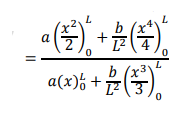$$(\frac{\frac{aL^{2}}{2}+\frac{bL^{2}}{4}}{aL+\frac{bL}{3}})$$

$$\frac{3L}{4}(\frac{2a+b}{3a+b})$$

14. A particle of mass m is projected with a speed u from the ground at angle is θ = π/3 w.r.t. horizontal (x-axis). When it has reached its maximum height, it collides completely inelastically with another particle of the same mass and velocity ̂

$$u\hat{i}$$
. The horizontal distance covered by the combined mass before reaching the ground is:

1. a.
$$\frac{3\sqrt{3}u^{2}}{8g}$$
2. b.
$$\frac{2\sqrt{2}u^{2}}{g}$$
3. c.
$$\frac{5u^{2}}{8g}$$
4. d.
$$\frac{3\sqrt{2}u^{2}}{4g}$$

Solution:

1.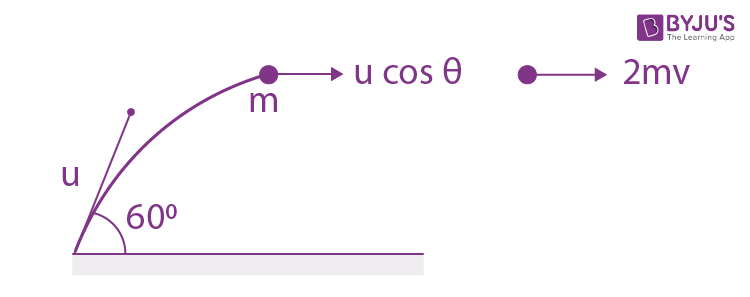The only external force acting on the colliding system during the collision is the gravitational force. Since gravitational force is non- impulsive, the linear momentum of the system is conserved just before and just after the collision.

pi = pf

mu+mucosθ =2mv

$$v=\frac{u(1+cos60^{0})}{2}=\frac{3}{4}u$$

So the horizontal range after the collision = vt

$$= v\sqrt{\frac{2H_{max}}{g}}$$

$$= \frac{3}{4}u\sqrt{\frac{2u^{2}sin^{2}60^{0}}{2g^{2}}}$$

$$= \frac{3}{4}u^{2}\frac{\sqrt{\frac{3}{4}}}{g}$$

$$\frac{3\sqrt{3}u^{2}}{8g}$$

15. A uniformly thick wheel with moment of inertia I and radius R is free to rotate about its center of mass (see fig). A massless string is wrapped over its rim and two blocks of massless string is wrapped over its rim and two blocks of masses m1 and m2 (m1>m2 ) are attached to the ends of string. The system is released from rest. The angular speed of the wheel when m1 descend by a distance h is: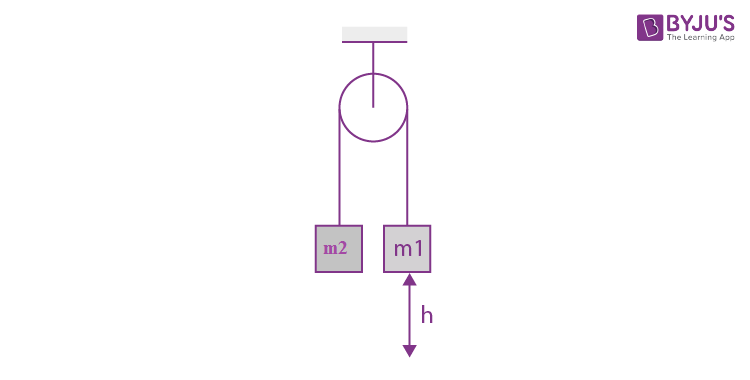1. a.
$$\frac{1}{R}\sqrt{\frac{2(m_{1}-m_{2})gh}{(m_{1}+m_{2})+\frac{1}{R^{2}}}}$$
2. b.
$$\frac{gh}{R}\sqrt{\frac{(m_{1}+m_{2})}{(m_{1}+m_{2})+\frac{1}{R^{4}}}}$$
3. c.
$$\frac{gh}{R}\sqrt{\frac{(m_{1}-m_{2})}{(m_{1}+m_{2})+\frac{1}{R}}}$$
4. d.
$$\frac{1}{R}\sqrt{\frac{2(m_{2}+m_{1})gh}{(m_{1}+m_{2})+\frac{1}{R^{2}}}}$$

Solution:

1. Assume initial potential energy of the blocks to be zero. Initial kinetic energy is also zero since the blocks are at rest.

When block m1 falls by h, m2 goes up by h (because of length constraint)

Final P.E = m2gh – m1gh

Let the final speed of the blocks be and angular velocity of the pulley be

Final K.E =

$$=\frac{1}{2}(m_{1}+m_{2})v^{2}+\frac{1}{2}I\omega ^{2}$$

Total energy is conserved. Hence,

$$0 =m_{2}gh-m_{1}gh+\frac{1}{2}(m_{1}+m_{2})v^{2}+\frac{1}{2}I\omega ^{2}$$

$$v=\omega r$$
(due to no slip condition)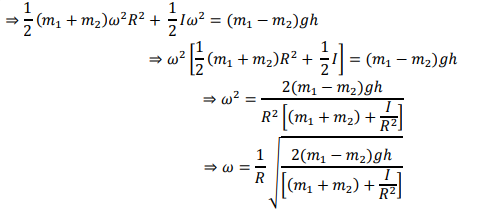Solution: (a)

16. The energy required to ionise a hydrogen like ion in its ground state is 9 Rydbergs. What is the wavelength of the radiation emitted when the electron in this ion jumps from the second excited state to the ground stare?

1. a. 8.6
2. b.11.4
3. c.24.2
4. d.35.8

Solution:

1. $$\frac{hc}{\lambda }=(13.6eV)Z^{2}(\frac{1}{n_{1}^{2}}-\frac{1}{n_{2}^{2}})$$

n1=1

n2= 3

For an H-like atom, ionization energy is (R)Z2.

This gives Z=3

$$\frac{hc}{\lambda }=(13.6eV)(3^{2})(\frac{1}{1^{2}}-\frac{1}{3^{2}})$$

Wavelength

$$\lambda =11.4nm$$

17. There is a small source of light at some depth below the surface of water (refractive index 4/3) in a tank of large cross sectional surface area. Neglecting any reflection from the bottom and absorption by water, percentage of light that emerges out of surface is (nearly): [Use the fact that surface area of a spherical cap of height h and radius of curvature r is 2πrh]

1. a.17%
2. b.34%
3. c.50%
4. d.21%

Solution:

1.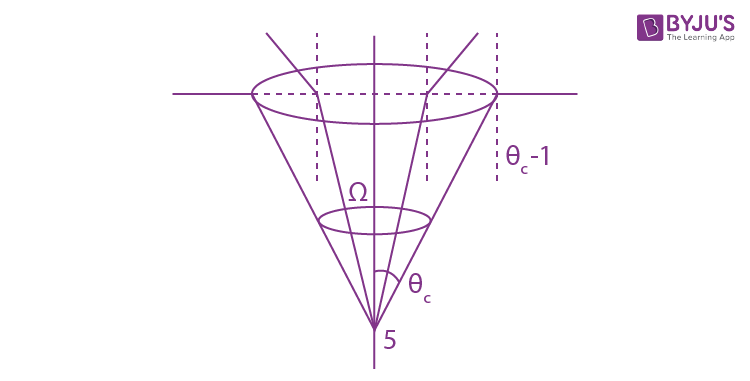The portion of light escaping into the air from the liquid will form a cone. As long as the angle of incidence on the liquid – air interface is less than the critical angle, i.e.i< θc , the light rays will undergo refraction and emerge into the air.

For i> θc , the light rays will suffer TIR. So, these rays will not emerge into the air. The portion of light rays emerging into the air from the liquid will form a cone of half angle = θc

sin θc = (1/μLiq) =3/4

cos θc =√7/4

Solid angle contained in this cone is

$$\Omega = 2\pi (1-cos\theta _{c})$$

Percentage of light that escapes from liquid =

$$(\Omega/4\pi)\times 100$$

Putting values we get

Percentage = ((4-√7)/8) x 100% = 17%

18. An electron of mass m and magnitude of charge |e| initially at rest gets accelerated by a constant electric field E. The of charge of de-Broglie wavelength of this electron at time t ignoring relativistic effects is

1. a.
$$-\frac{\left | e \right |Et}{h}$$
2. b.
$$-\frac{h}{\left | e \right |E\sqrt{t}}$$
3. c.
$$-\frac{h}{\left | e \right |Et^{2}}$$
4. d.
$$-\frac{2ht^{2}}{\left | e \right |E}$$

Solution:

1.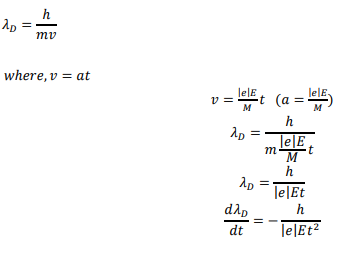19. In LC circuit the inductance L = 40mH and C = 100 μF. If a voltage V(t) = 10sin(314t) is applied to the circuit, the current in the circuit is given as

1. a. 10cos (314t)
2. b. 0.52cos (314t)
3. c. 0.52sin (314t)
4. d. 5.2cos (314t)

Solution:

1.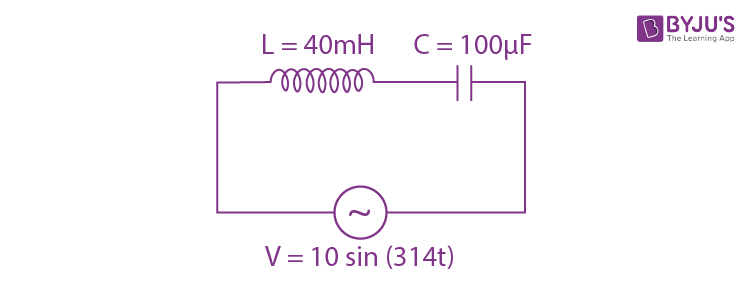$$Impedance Z =\sqrt{R^{2}+(X_{c}-X_{L})^{2}}$$

$$=\sqrt{(X_{c}-X_{L})^{2}}$$

R = 0

= XC - XL

= (1/ωC) – ωL

= [(1/314 x 100 x 10-6)] – (314 x 40 x 10-3)

= 31.84 – 12.56 = 19.28 ohm

For XC >XL, current leads voltage by π/2

$$i=\frac{V}{Z}=\frac{10sin(314t+\frac{\pi }{2})}{19.28}$$

= 0.52cos(314t)

20. The current (i) in the network is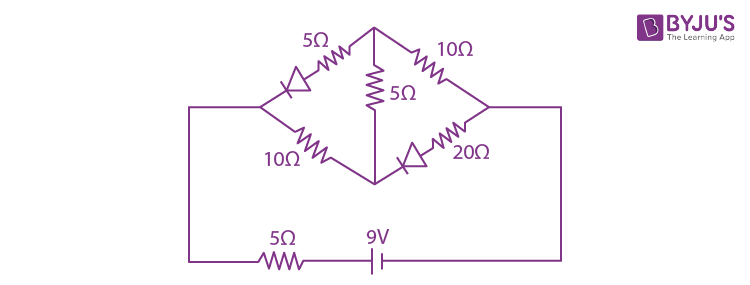1. a. 0A
2. b. 0.3 A
3. c. 0.2 A
4. d. 0.6 A

Solution:

1. Since the diodes are reverse biased, they will not conduct. Hence, the circuit will look like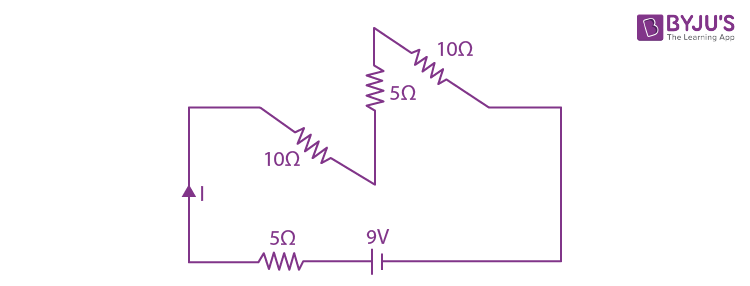Reff = 5+10+5+10 = 30 ohm

I = 9/30 = 0.3A

21. Starting at temperature 300 K, one mole of an ideal diatomic gas (ϒ = 1.4) is first compressed adiabatically from volume to V1 to V2 = V1/16. It is then allowed to expand isobarically to volume 2V2 . If all the processes are the quasi-static then the final temperature of the gas (0K) is (to the nearest integer)

Solution:

1.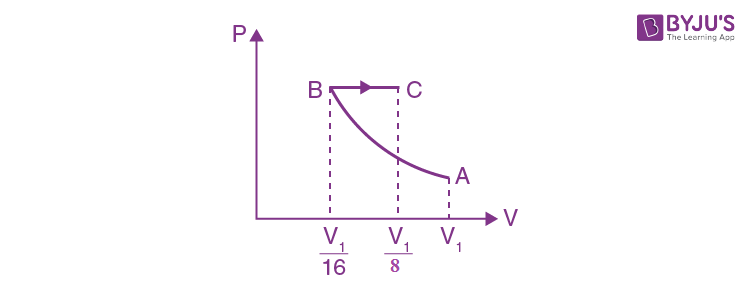$$PV^{\gamma }=constant$$

$$TV^{\gamma-1 }=constant$$

300(V1) (1.4 -1) = TB(V1/16)2/5

TB = 300 x 2(8/5)

Now for BC process

(VB/VC) = (VC/TC)

$$T_{C}=\frac{V_{C}T_{B}}{T_{B}}=2\times 300\times 2^{8/5}$$

TC= 1818 K

22. An electric field

$$\vec{E}=4x\hat{i}-(y^{2}+1)\hat{j}$$
passes through the box shown in figure. The flux of the electric field through surface ABCD and BCGF are marked as
$$\phi _{1}$$
and
$$\phi _{2}$$
, then difference between
$$\phi _{1}-\phi _{2}$$
is (Nm2/C )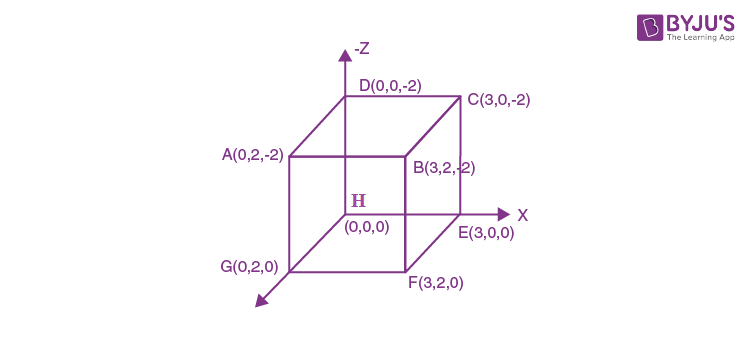Solution:

1. Electric flux through a surface is

$$\phi = \int \vec{E}=d\vec{A}$$

For surface ABCD

$$d\vec{A}$$
is along
$$-\hat{k}$$

So, at all the points of this surface,

$$\vec{E}.d\vec{A}=0$$

Because,

$$\phi _{ABCD}=\phi _{1}=0$$

For surface BCEF

$$d\vec{A}$$
is along
$$\hat{i}$$

$$\vec{E}.d\vec{A}=E_{x}dA$$

$$\phi _{BCEF}=\phi _{2}=4x(2\times 2)$$

If x = 3

$$\phi _{2}=48\frac{Nm^{2}}{C}$$

$$\phi _{1}-\phi _{2}=-48\frac{Nm^{2}}{C}$$

23. In a Young’s double slit experiment 15 fringes are observed on a small portion of the screen when light of wavelength 500nm is used. 10 fringes are observed on the same section of the screen when another light source of wavelength λ is used. Then the value of λ is (nm)

Solution:

1. If the length of the segment is y,

Then y = nβ

n= no.of fringes

β = frindge width

15 x 500 x (D/d) = 10 x λ2 x (D/d)

λ2 = 15 x 50 nm

= 750 nm

24. In a meter bridge experiment S is a standard resistance. R is a resistance wire. It is found that balancing length is l = 25 cm. If R is replaced by a wire of half length and half diameter that of R of same material, then the balancing l (in cm) will now be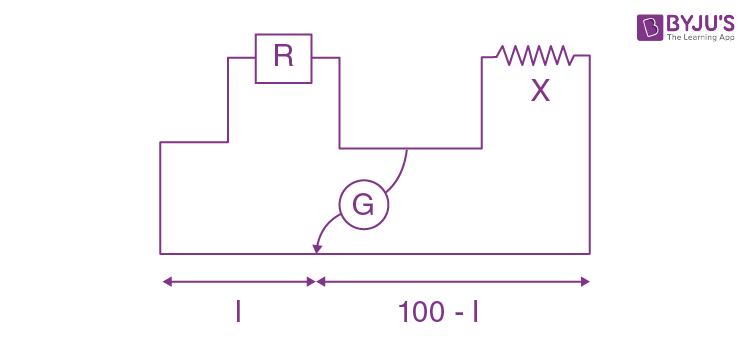Solution:

1. (X/R) = (75/25) = 3

$$R = \frac{\rho l}{A}=\frac{4\rho l}{\pi d^{2}}$$

$$R^{'} = \frac{4\rho \frac{l}{2}}{\pi (d/2)^{2}}= 2R$$

Then

$$\frac{X}{R^{'}} = \frac{100-l}{l}$$

$$\frac{X}{2R} = \frac{100-l}{l}=\frac{3}{2}$$

l= 40.00 cm

25. The circuit shown below is working as a 8V dc regulated voltage source. When 12V is used as input, the power dissipated (in mW) in each diode id; (considering both zener diode are identical).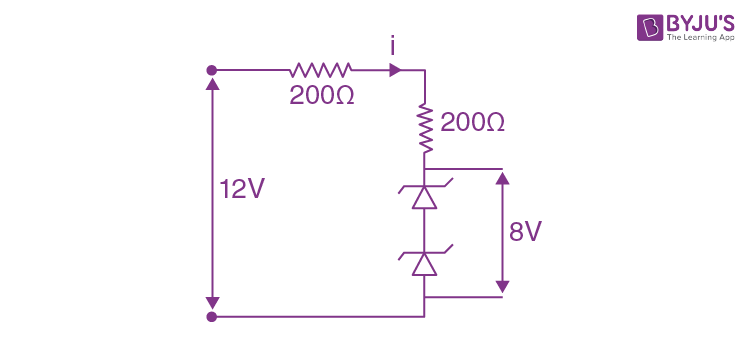Solution:

1. I=[(12-8)/(200+200)]A = 4/400 = 10-2 A

Power loss in each diode = 4(10-2) W = 40 mW

### JEE Main 2020 Physics Paper January 9 Shift 2